# Repetition Periods for Elementary Cellular Automata

A collection of rules and their repetition periods as a function of size

Originator: Stephen Wolfram

A plot of these repetition periods as originally provided on page 260 of A New Kind of Science (Wolfram, 2002) and a visualization was provided on page 259

(2 columns, 5 rows)

## Examples

### Basic Examples

Retrieve the ResourceObject:

 In:=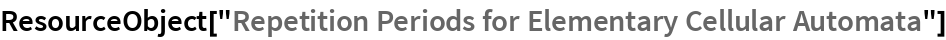Out=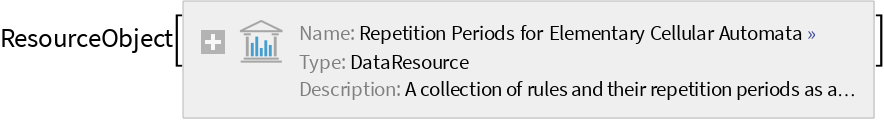View the data:

 In:=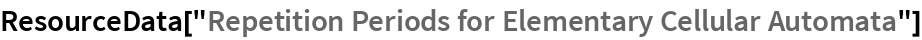Out=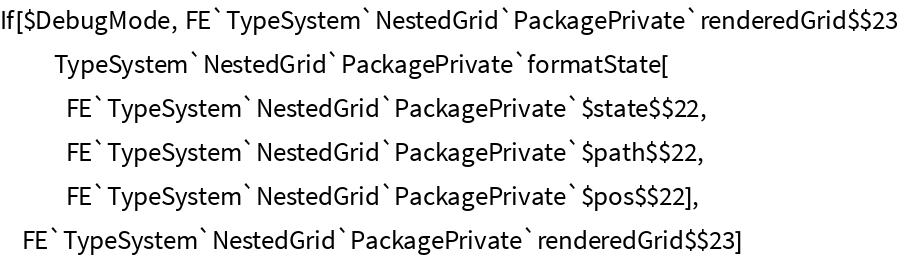### Visualization

Find the systems provided:

 In:=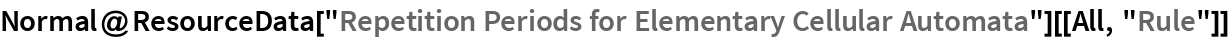Out=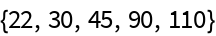Find the systems sizes for rule 90 with repetition period between 5 and 50:

 In:=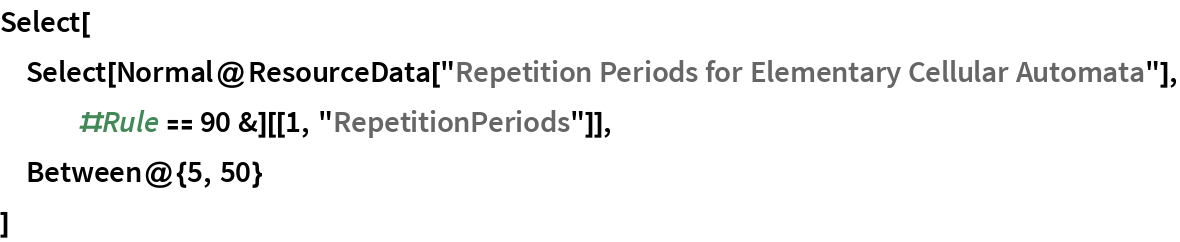Out=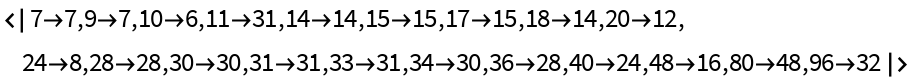Plot these:

 In:=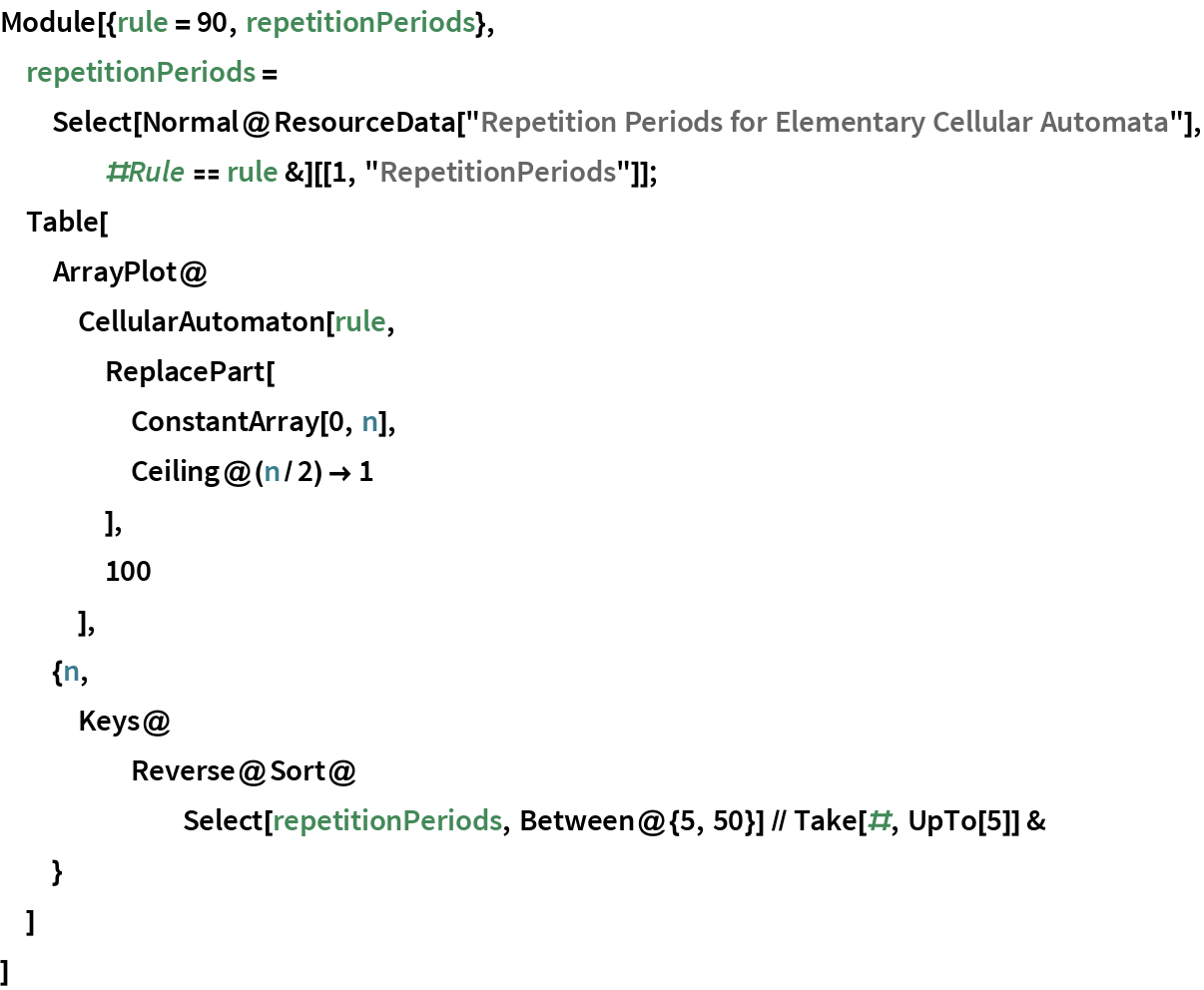Out=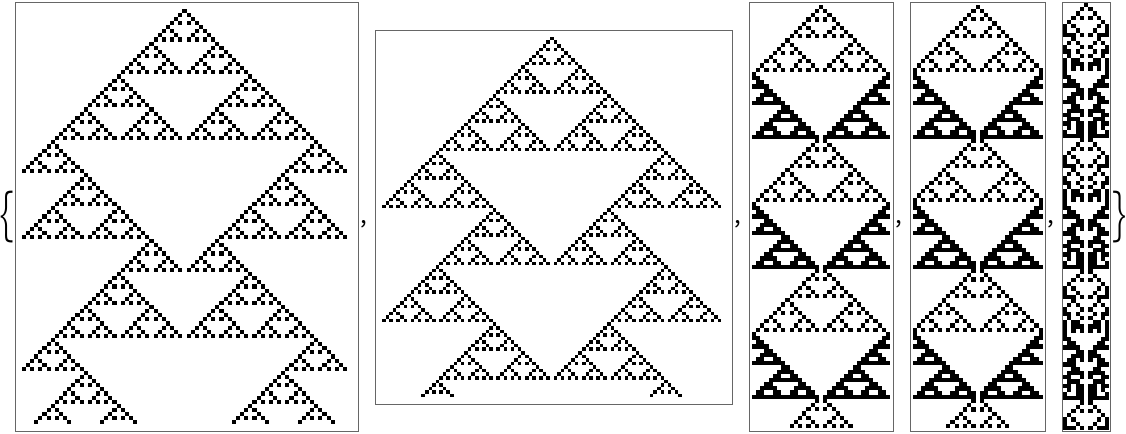Highlight the repeating blocks:

 In:=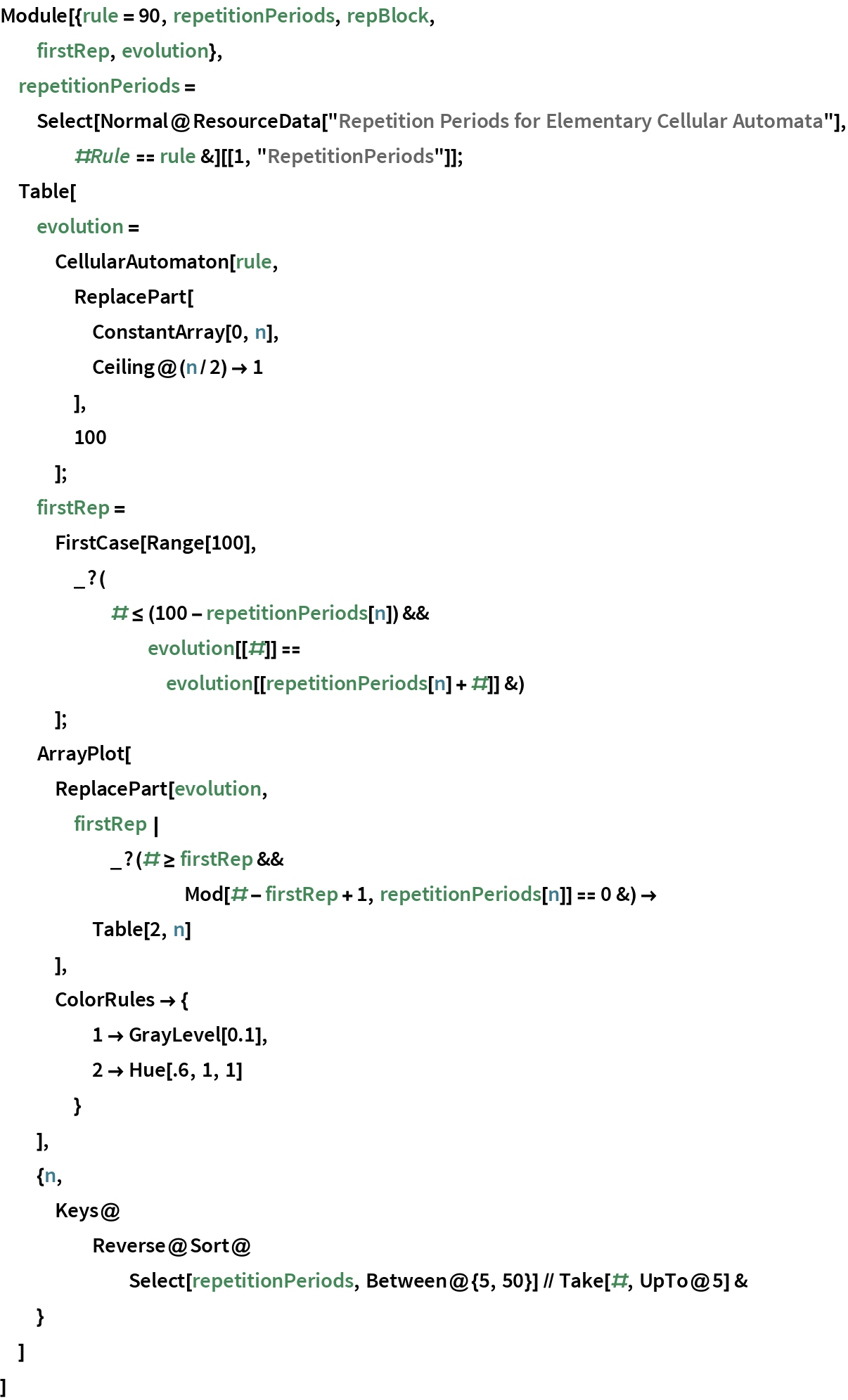Out=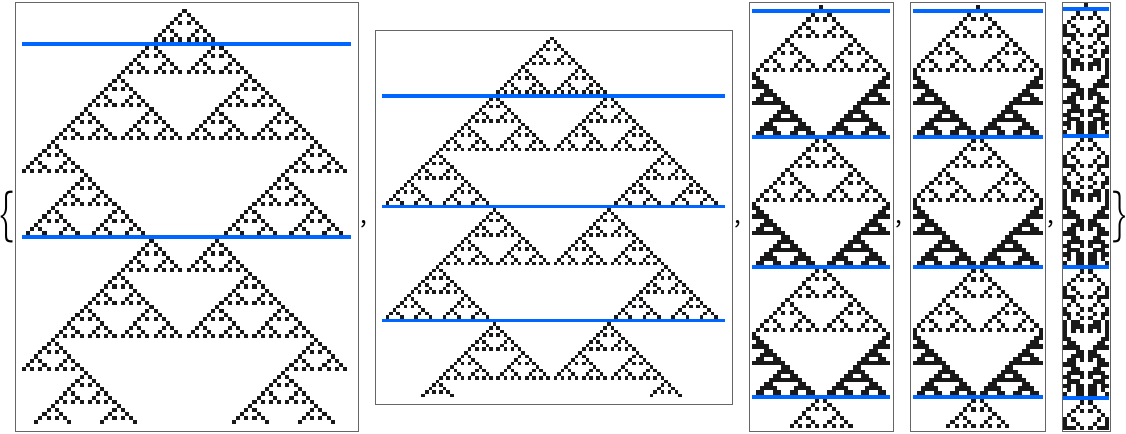Wolfram Research, "Repetition Periods for Elementary Cellular Automata" from the Wolfram Data Repository (2017)